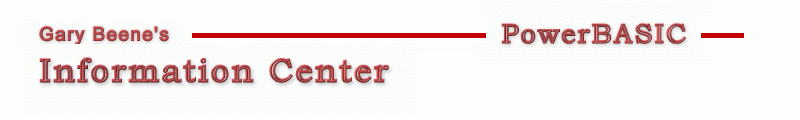## Randomly Shuffle

Category: Arrays

Date: 03-28-2012

'Shuffling is accomplished by cycling through the array, exchanging
'each element with a randomly selected element in the array.

'Primary Code:
'written as a function
Sub ShuffleArray(TheArray() As Long)
Dim L as Long, U as Long, i As Long, j as Long
Randomize Timer :  L = LBound(TheArray) : U = UBound(TheArray)
For i = L to U : j = Rnd(L,U) :  Swap TheArray(i), TheArray(j) : Next i
End Sub

'Compilable Example:
'this example walks through an array, putting its values in the caption of the dialog
#Compiler PBWin 9, PBWin 10
#Compile EXE
#Dim All
#Include "Win32API.inc"
Global hDlg As Dword

Function PBMain() As Long
Dialog New Pixels, 0, "Array Shuffle Test Code",300,300,240,200, %WS_OverlappedWindow To hDlg
Control Add Button, hDlg, 100, "Shuffle Array", 30,10,130,25
Dialog Show Modal hDlg Call DlgProc
End Function

CallBack Function DlgProc() As Long
If CB.Msg = %WM_Command AND CB.Ctl = 100 AND CB.Ctlmsg = %BN_Clicked Then
'create array
Dim MyArray(5) as Long, i as Long, temp\$
Array Assign MyArray() = 0,1,2,3,4,5  'sample data
'shuffle it
ShuffleArray MyArray()
temp\$ = "Array now contains: " + \$crlf + \$crlf + Str\$(myarray(0))
For i = 1 To 5 : temp\$ = temp\$ + " - " + Str\$(myarray(i)) : Next i
MsgBox temp\$
End If
End Function

Sub ShuffleArray(TheArray() As Long)
Dim L as Long, U as Long, i As Long, j as Long
Randomize Timer :  L = LBound(TheArray) : U = UBound(TheArray)
For i = L to U : j = Rnd(L,U) :  Swap TheArray(i), TheArray(j) : Next i
End Sub

'gbs_00069
'Date: 03-10-2012

created by gbSnippets
http://www.garybeene.com/sw/gbsnippets.htm